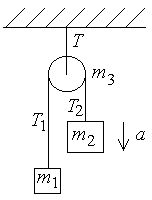First Name: ___________________ Last Name: ____________________ Section: _________

December 17, 1999 Physics 207

Final Exam

Print your name and section clearly on all nine pages. (If you do not know your section number, write your TAs name.) Show all work in the space immediately below each problem. Your final answer must be placed in the box provided. Problems will be graded on reasoning and intermediate steps as well as on the final answer. Be sure to include units wherever necessary, and the direction of vectors. Each problem is worth 25 points. In doing the problems, try to be neat. Check your answers to see that they have the correct dimensions (units) and are the right order of magnitudes. You are allowed two 8½ x 11" sheets of notes and no other references. The exam lasts exactly 2 hours.

(Do not write below)

SCORE:

Problem 1: __________

Problem 2: __________

Problem 3: __________

Problem 4: __________

Problem 5: __________

Problem 6: __________

Problem 7: __________

Problem 8: __________

TOTAL: ___________

First Name: ___________________ Last Name: ____________________ Section: _________

1. An object released from rest at a height of 5 m above the ground hits the ground with a speed of 7 m/s.

a. What fraction of the initial potential energy of the object is lost to friction with the air? (9 pts.)

b. If the collision with the ground is perfectly elastic and results in an impulse of 70 kg-m/s, what is the mass of the object? (7 pts.)

c. If the collision with the ground is perfectly elastic and the object loses the same fraction of its mechanical energy between each bounce, what is its speed just before it hits the ground the second time? (9 pts.)

First Name: ___________________ Last Name: ____________________ Section: _________

2. A wheel of mass 20 kg and radius 0.5 m initially at rest rolls down a hill 10 m high without slipping and without losing mechanical energy. It reaches the bottom with a speed of 13 m/s.

a. What is the moment of inertia of the wheel? (15 pts.)

b. What fraction of the kinetic energy is in rotation? (10 pts.)

First Name: ___________________ Last Name: ____________________ Section: _________

3. Two masses, m1 = 18 kg and m2 = 28 kg, initially at rest are suspended from a pulley with massless ropes as shown. The pulley has a mass of m3 = 3 kg concentrated at its rim.a. What is the acceleration of the mass m2? (15 pts.)

b. What is the tension T in the upper rope? (10 pts.)

First Name: ___________________ Last Name: ____________________ Section: _________

4. An automobile is traveling around a curve in a road with a radius of curvature of 50 m banked at an angle of 45º

a. What is the maximum speed for which the car will not slip sideways, assuming a coefficient of friction of the tires with the road of 0.5? (15 pts.)

b. What coefficient of static friction is required for the car to remain parked on the banked road? (10 pts.)

First Name: ___________________ Last Name: ____________________ Section: _________

5. A cube, 20 cm on a side has a uniform density of 750 kg/m3 and floats on the surface of a calm body of water.

a. What fraction of the cubes volume is above the level of the water? (10 pts.)

b. At what angular frequency w will the cube bob up and down in the water if it is initially displaced slightly vertically from its equilibrium position? (15 pts.)

First Name: ___________________ Last Name: ____________________ Section: _________

6. A string with a length of 50 cm and mass of 10 g is fixed at both ends and has a tension of 450 N.

a. What is the frequency of its lowest mode of transverse vibration? (10 pts.)

b. At what distance from the end of the string is the first node if the string is vibrating at four times its fundamental frequency? (5 pts.)

c. By what factor should the amplitude of the string vibration be increased to produce a 3 dB increase in the sound intensity? (10 pts.)

First Name: ___________________ Last Name: ____________________ Section: _________

7. A sonar determines the depth of an object beneath the water by measuring the time required for a sound to travel to the object and be reflected back. The velocity of sound in water is 1500 m/s.

a. What is the depth of an object for which the reflected sound returns in 40 ms? (8 pts.)

b. Assuming the object is solid with a large bulk modulus, what is the lowest frequency for which the reflected wave above will destructively interfere with the transmitted wave at the source? (8 pts.)

c. If the object is moving upward at a velocity of 1 m/s, at what transmitted frequency will the reflected wave produce a beat frequency of 1 Hz with the transmitted wave? (9 pts.)

First Name: ___________________ Last Name: ____________________ Section: _________

8. One hundred moles of an ideal, monotomic gas as a temperature of 27° C is contained in a volume of 0.1 m3.

a. What is the pressure of the gas? (6 pts.)

b. If the speed of sound in the gas is 1020 m/s, what is the molecular weight of the gas? (6 pts.)

c. If 25 kJ of heat is added to the gas at constant volume, by how much does its temperature rise? (7 pts.)

d. What is the change in entropy of the gas for the above process? (6 pts.)